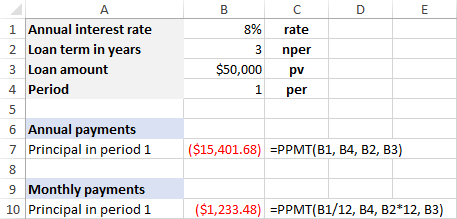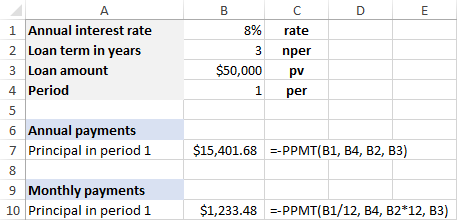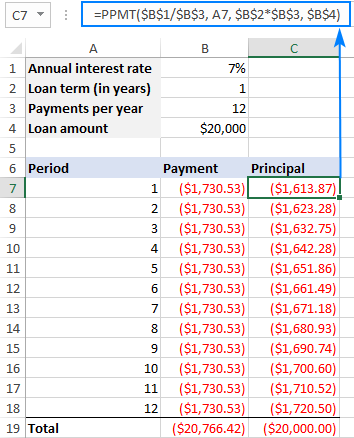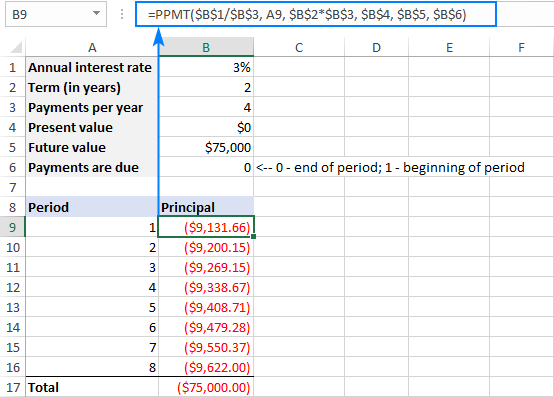# Excel PPMT function with formula examples

The tutorial shows how to use the PPMT function in Excel to calculate the payment on the principal for a loan or investment.

When you make periodic payments on a loan or mortgage, a certain part of each payment goes towards the interest (fee charged for borrowing) and the remainder of the payment goes to paying off the loan principal (the amount you originally borrowed). While the total payment amount is constant for all periods, the principal and interest parts are different - with each succeeding payment less is applied to the interest and more to the principal.

Microsoft Excel has special functions to find both the total payment amount and its parts. In this tutorial, we will look at how to use the PPMT function to calculate the payment on the principal.

## Excel PPMT function - syntax and basic uses

The PPMT function in Excel calculates the principal portion of a loan payment for a given period based on a constant interest rate and payment schedule.

The syntax of the PPMT function is as follows:

PPMT(rate, per, nper, pv, [fv], [type])

Where:

• Rate (required) - the constant interest rate for the loan. Can be provided as percentage or a decimal number.

For example, if you make annual payments on a loan or investment with an annual interest rate of 7 percent, supply 7% or 0.07. If you make monthly payments on the same loan, then supply 7%/12.

• Per (required) - the target payment period. It should be an integer between 1 and nper.
• Nper (required) - the total number of payments for the loan or investment.
• Pv (required) - the present value, i.e. how much a series of future payments is worth now. The present value of a loan is the amount you originally borrowed.
• Fv (optional) - the future value, i.e. the balance you wish to have after the last payment is made. If omitted, it is assumed to be zero (0).
• Type (optional) - indicates when the payments are due:
• 0 or omitted - payments are due at the end of each period.
• 1 - payments are due at the beginning of each period.

For example, if you borrow \$50,000 for 3 years with an annual interest rate of 8% and you make annual payments, the following formula will calculate the principal portion of a loan payment for period 1:

`=PPMT(8%, 1, 3, 50000)`

If you are going to make monthly payments on the same loan, then use this formula:

`=PPMT(8%/12, 1, 3*12, 50000)`

Instead of hardcoding the arguments in the formula, you can input them in the predefined cells and refer to those cells like shown in this screenshot:If you prefer to have the result as a positive number, then put a minus sign before either the entire PPMT formula or the pv argument (loan amount). For example:

`=-PPMT(8%, 1, 3, 50000)`

or

`=PPMT(8%, 1, 3, -50000)`### 3 things you should know about Excel PPMT function

To successfully use PPMT formulas in your worksheets, please keep in mind the following facts:

1. The principal is returned as a negative number because it is an outgoing payment.
2. By default, the Currency format is applied to the result, with negative numbers highlighted in red and enclosed in parenthesis.
3. When calculating the principal amount for different payment frequencies, be sure you are consistent with the rate and nper arguments. For rate, divide the annual interest rate by the number of payments per year (assuming it is equal to the number of compounding periods per year). For nper, multiply the number of years by the number of payments per year.
• weeks: rate - annual interest rate/52; nper - years*52
• months: rate - annual interest rate/12; nper - years*12
• quarters: rate - annual interest rate/4; nper - years*4

## Examples of using PPMT formula in Excel

And now, let's take a couple of formula examples that show how to use the PPMT function in Excel.

### Example 1. Short form of PPMT formula

Supposing, you want to calculate the payments on the principal for a loan. In this example, that will be 12 monthly payments, but the same formula will work for other payment frequencies as well such as weekly, quarterly, semi-annual or annual.

To save you the trouble of writing a different formula for each period, enter the period numbers in some cells, say A7:A18, and set up the following input cells:

• B1 - annual interest rate
• B2 - loan term (in years)
• B3 - number of payments per year
• B4 - loan amount

Based on the input cells, define the arguments for your PPMT formula:

• Rate - annual interest rate / the number of payments per year (\$B\$1/\$B\$3).
• Per - first payment period (A7).
• Nper - years * the number of payments per year (\$B\$2*\$B\$3).
• Pv - the loan amount (\$B\$4)
• Fv - omitted, assuming zero balance after the last payment.
• Type - omitted, assuming payments are due at the end of each period.

Now, put all the arguments together and you will get the following formula:

`=PPMT(\$B\$1/\$B\$3, A7, \$B\$2*\$B\$3, \$B\$4)`

Please pay attention, that we use absolute cell references in all the arguments except per where a relative cell reference (A7) is used. This is because the rate, nper and pv arguments refer to the input cells and should remain constant no matter where the formula is copied. The per argument should change based on the relative position of a row.

Enter the above formula in C7, then drag it down to as many cells as needed, and you will get the following result:As you can see in the screenshot above, the total payment (calculated with the PMT function) is the same for all the periods while the principal portion increases with each successive period because initially more interest than principal is paid.

To verify the results of the PPMT function, you can add up all the principal payments by using the SUM function, and see if the sum equals the original loan amount, which is \$20,000 in our case.

### Example 2. Full form of PPMT formula

For this example, we will utilize the PPMT function to calculate the payments on the principal required to increase an investment from \$0 to the amount you specify.

Since we are going to use the full form of the PPMT function, we define more input cells:

• B1 - annual interest rate
• B2 - investment term in years
• B3 - number of payments per year
• B4 - the present value (pv)
• B5 - the future value (fv)
• B6 - when the payments are due (type)

As with the previous example, for rate, we divide the annual interest rate by the number of payments per year (\$B\$1/\$B\$3). For nper, we multiply the number of years by the number of payments per year (\$B\$2*\$B\$3).

With the first payment period number in A10, the formula takes the following shape:

`=PPMT(\$B\$1/\$B\$3, A10, \$B\$2*\$B\$3, \$B\$4, \$B\$5, \$B\$7)`

In this example, the payments are made at the end of each quarter over a period of 2 years. Please notice that the sum of all principal payments equals the future value of the investment:## Excel PPMT function not working

If a PPMT formula is not working correctly in your worksheet, these troubleshooting tips may help:

1. The per argument should be greater than 0 but less than or equal to nper, otherwise a #NUM! error occurs.
2. All the arguments should be numeric, otherwise a #VALUE! error occurs.
3. When calculating weekly, monthly or quarterly payments, be sure to convert an annual interest rate to the corresponding period rate as shown in the above examples, otherwise the result of your PPMT formula will be wrong.

That's how you use the PPMT function in Excel. To get some practice, you are welcome to download our PPMT Formula Examples. I thank you for reading and hope to see you on our blog next week!